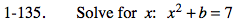### Home > PC > Chapter 1 > Lesson 1.4.1 > Problem1-135

1-135.

Solve for x: x2 + b = 7 Homework Help ✎Subtract b from both sides of the equation.

$\textit{x}^2= 7 - b$

Take the square root of both sides.

$\textit{x}=\pm\sqrt{7-b}$